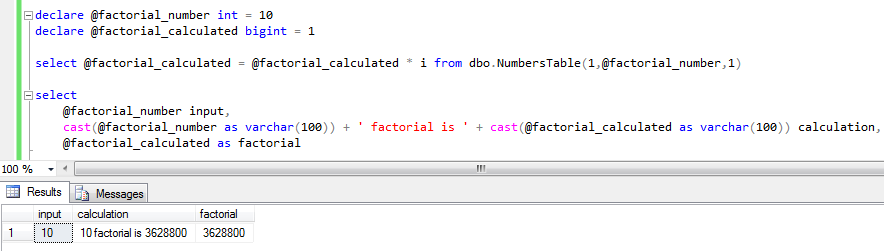﻿ SQL Factorial Function to Calculate Factorial of an Integer in SQL ServerSQL Server and T-SQL Development Tutorials Development resources, articles, tutorials, code samples, tools and downloads for ASP.Net, SQL Server, Reporting Services, T-SQL, Windows, AWS, SAP HANA and ABAP

# SQL Factorial Function to Calculate Factorial of an Integer in SQL Server

In SQL Server, developers can use SQL factorial function given in this tutorial to calculate factorial for a given integer value. For mathematical factorial calculation of an integer input value in SQL Server, sqlFactorial() user-defined function can be used as shown in the SQL tutorial.

If you don't already have a numbers table in your database, you can use following SQL codes to calculate factorial value of an input integer value.

Let's see the resultsOn the other hand, if you have a numbers table in your SQL Server database the above code can be converted into a more readable SQL script as follows:

SQL programmers can check SQL tutorial Create Numbers Table in SQL Server for dbo.NumbersTable() table valued function codes

Here is the output for 10! calculation (10 factorial calculation) in SQL Server for programmers.Of course it is easier to convert the second method into a SQL factorial function to calculate factorial values easily.

Here is how SQL programmers can use sqlFactorial user-defined function (UDF) to calculate factorial value of an input positive integer value.SQL Server